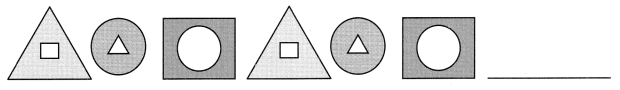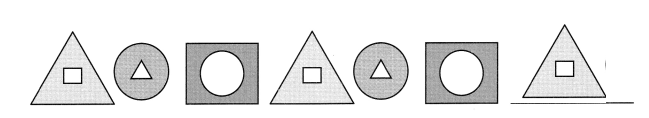# Math in Focus Grade 2 Chapter 18 Answer Key Lines and Surfaces

This handy Math in Focus Grade 2 Workbook Answer Key Chapter 18 Lines and Surfaces detailed solutions for the textbook questions.

## Math in Focus Grade 2 Chapter 18 Answer Key Lines and Surfaces

Math Journal

Draw a happy face using curves only.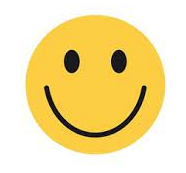Explanation:
I used curves to draw a happy face.

Draw a sad face using parts of lines and curves.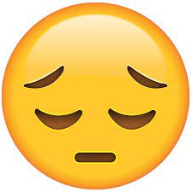Explanation:
I used parts of lines and curves to draw a sad face.

Challenging practice

The shapes at the bottom of this page can be combined to make a square. Color the pieces yellow. Cut them out and paste them below to make the square.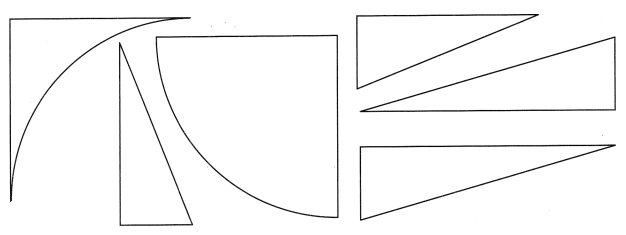Chapter Review/Test

Vocabulary

Fill in the blanks with words from the box.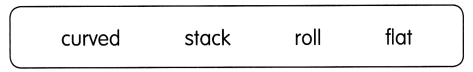Question 1.
A ball has a __________ surface.
It can _________ on the ground.
A ball has a curved surface.
It can roll on the ground.

Question 2.
A picture has a __________ surface.
You can ___________ pictures on top of each other.
A picture has a flat surface.
You can stack pictures on top of each other.

Concepts and Skills

Question 3.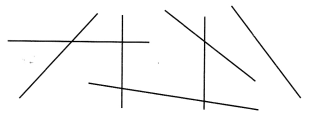These are (parts of lines, curves).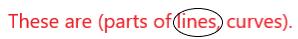Explanation:
All the above shapes are parts of lines.

Question 4.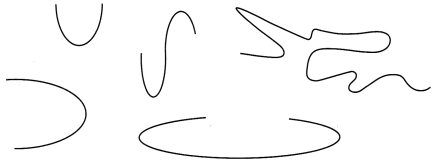These are (parts of lines, curves).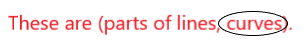Explanation:
All the above shapes are curves.

Draw

Question 5.
A figure that has five parts of lines and three curves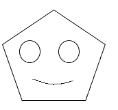Explanation:
The figure has five parts of lines and three curves.

Question 6.
A figure that has only parts of lines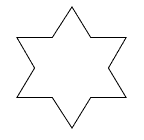Explanation:
The above figure has only parts of lines.

Question 7.
An object that has only curved surfaces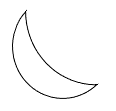Explanation:
The above object has only curved surfaces.

Question 8.
An object that has two flat surfaces and one curved surface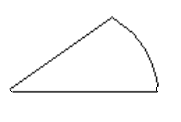Explanation:
The above object has 2 flat surfaces and 1 one curved surface.

Problem Solving

Each pattern is made of curves and straight lines. Find the pattern. Then complete the pattern.

Question 9.